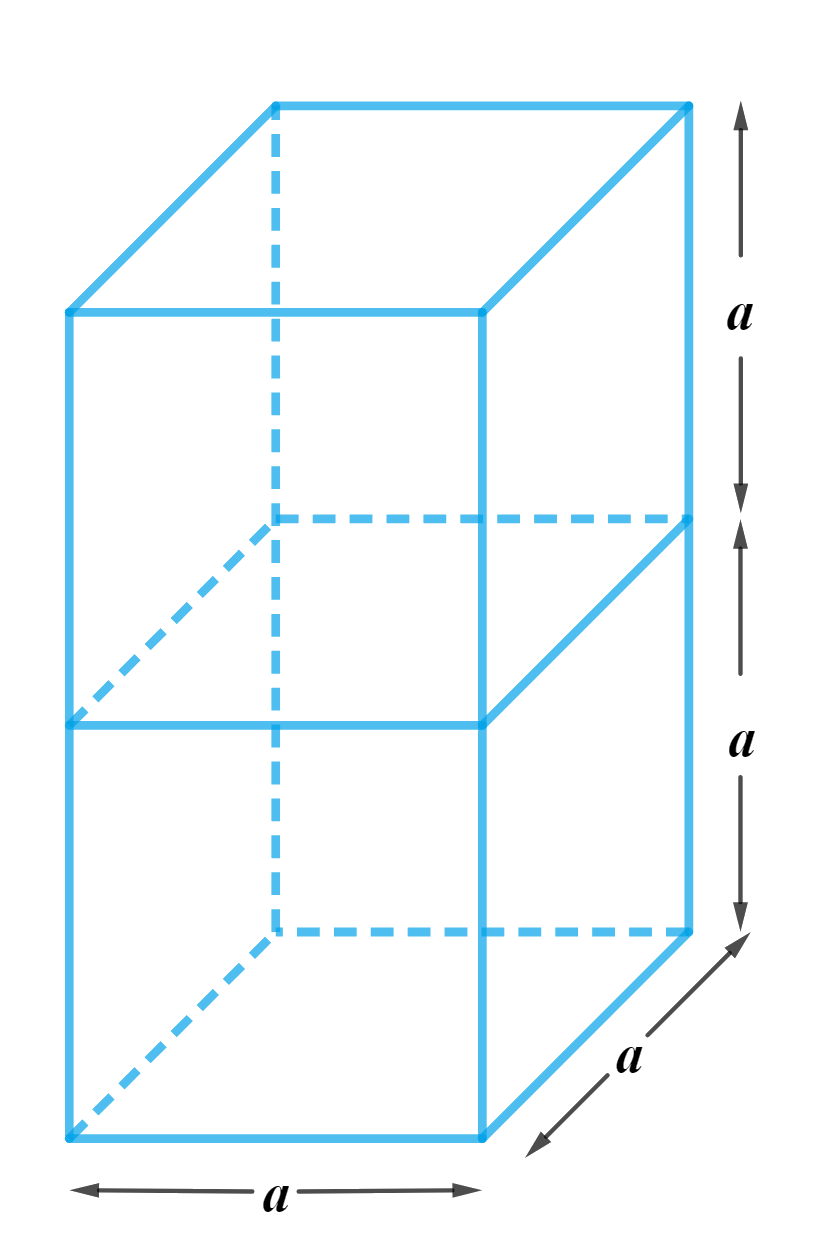# Ex.13.1 Q1 Surface Areas and Volumes Solution - NCERT Maths Class 10

Go back to  'Ex.13.1'

## Question

$$2$$ cubes each of volume $$64 \;\rm{cm}^3$$ are joined end to end. Find the surface area of the resulting cuboid.

## Text Solution

What is known?

Two cubes each of volume $$64 \;\rm{cm}^3$$  are joined end to end.

What is unknown?

Surface area of the resulting cuboid when two cubes are joined end to end.

Reasoning:

We will find the length of the edge of each cube by using the formula for volume of a cube $$=a^3$$ , where length of the edge is $$a.$$

As the cubes are joined end to end, they will appear as followsUsing the formula for Surface area of a cuboid \begin{align} = 2\left( {lb + bh + lh} \right)\end{align} ,

where $$l,b\,\,{\rm{ and }}\,\,h$$  are length, breadth and height respectively. We’ll be able to get the answer.

Steps:

Let the length of the edge of each cube is $$a$$
Therefore, volume of the cube $$=a^3$$

\begin{align}\text{volume of the cube,} {a^3} &= 64{c{m^3}}\\{a^3} &= 64{c{m^3}}\\a &= \sqrt{{64c{m^3}}}\\a &= \sqrt{{{{\left( {4{cm}} \right)}^3}}}\\a &= 4{cm}\end{align}

Therefore,

Length of the resulting cuboid,$$l = a = 4\rm{cm}$$
Breadth of the resulting cuboid,$$b = a = 4\rm{cm}$$
Height of the resulting cuboid,$$h = 2a = 2 \times 4\rm{cm} = 8\rm{cm}$$

Surface area of the resulting cuboid $$= 2\left( {lb + bh + lh} \right)$$

\begin{align}&= 2\left( {4{cm} \times 4{cm} + 4{cm} \times 8{cm} + 4{cm} \times 8{cm}} \right)\\&= 2\left( {16{c{m^2}} + 32{c{m^2}} + 32{c{m^2}}} \right)\\\ &= 2 \times 80{c{m^2}}\\&= 160{c{m^2}}\end{align}

Learn from the best math teachers and top your exams

• Live one on one classroom and doubt clearing
• Practice worksheets in and after class for conceptual clarity
• Personalized curriculum to keep up with school